Thank you for visiting nature.com. You are using a browser version with limited support for CSS. To obtain the best experience, we recommend you use a more up to date browser (or turn off compatibility mode in Internet Explorer). In the meantime, to ensure continued support, we are displaying the site without styles and JavaScript.

# On the Origin of Protein Superfamilies and Superfolds

## Abstract

Distributions of protein families and folds in genomes are highly skewed, having a small number of prevalent superfamiles/superfolds and a large number of families/folds of a small size. Why are the distributions of protein families and folds skewed? Why are there only a limited number of protein families? Here, we employ an information theoretic approach to investigate the protein sequence-structure relationship that leads to the skewed distributions. We consider that protein sequences and folds constitute an information theoretic channel and computed the most efficient distribution of sequences that code all protein folds. The identified distributions of sequences and folds are found to follow a power law, consistent with those observed for proteins in nature. Importantly, the skewed distributions of sequences and folds are suggested to have different origins: the skewed distribution of sequences is due to evolutionary pressure to achieve efficient coding of necessary folds, whereas that of folds is based on the thermodynamic stability of folds. The current study provides a new information theoretic framework for proteins that could be widely applied for understanding protein sequences, structures, functions and interactions.

## Introduction

It is well known from observation of protein sequence and structure databases that distributions of protein sequences, families and folds are highly skewed1,2,3,4,5, having a small number of prevalent families (superfamilies) or folds (superfolds) and a large number of families/folds of a small size. Sequences of known proteins only utilize a small fraction of all the possible combinations of amino acid sequences6,7. Similarly, it is estimated that the number of protein folds is limited in nature6,7,8,9. The number of membrane proteins is also estimated to be limited8. Protein families and folds are typical examples of biological entities that have skewed, power-law distributions1. The discovery of superfamilies and superfolds as well as the skewness of the sequence and fold distributions is one of the important achievements of bioinformatics and network science in the past two decades. The origin of the skewed distribution of protein families and folds has long been discussed from various aspects: a mathematical evolution model was proposed, which explains the power-law distribution with gene duplications and acquisition of new genes through gene transfer1. Using a computational simulation on a protein model, superfamilies with thermodynamically stable folds were observed to emerge10,11. Finkelstein discussed that the number of protein folds is limited due to their topological constraints12. Why some protein sequence superfamilies and folds have many members and why their overall distributions are skewed are fundamental questions in molecular biology, evolution and bioinformatics. Explanations of the questions also have strong implications for experimental evolution and protein design.

Here, we employ an information theoretic approach13 to investigate the relationship between sequences and structures that leads to the skewed distribution of families and folds. Information theory deals with quantification of flow of information in communication. Following Anfinsen's postulate that a protein sequence encodes information of its tertiary structure14, we consider that protein sequences and folds constitute an information theoretic channel. A channel is a system which takes input signals (here protein sequences) and transfers them to produce output messages (here protein folds). To construct the channel, we used a lattice model of proteins, which allows enumeration of all possible sequences and folds as well as computation of the probability that each sequence folds into a particular fold. By computing the capacity of the channel, we obtained the most efficient distribution of sequences that code all protein folds. It is often said that biology is about information flow and one of the most fundamental information flows in biology is observed in translation of DNA to an amino acid sequence, which codes for a protein tertiary structure. However, to the best of our knowledge, this is the first time that the protein sequence-structure relationship has been investigated formally as an information theoretic channel. Lattice models have been frequently used for investigating physical aspects of protein sequences and folds15,16; but this is the first time that the protein sequence-structure relationship is examined as the entire population of proteins in an organism.

The identified distribution of sequences and folds are found to follow a power law, consistent with those observed for proteins in nature. These results suggest that underlying evolutionary pressure for protein sequences includes efficient coding of protein folds. Importantly, the skewed distributions of sequences and folds are suggested to have different origins: the skewed distribution of sequences is due to evolutionary pressure to achieve efficient coding of necessary folds, whereas that of folds is based on the thermodynamics of folds. Close investigation found that, consistent with previous works15,16, highly populated sequences tend to code a single fold that has a distinctively low energy compared to the other folds, whereas the least populated sequences code multiple unstable folds with an equal, small probability. Thus, the current work uniformly explains the behavior of protein sequences and folds as the entire population as well as energetic characteristics of populated and less populated individual proteins.

## Results

### Protein sequence-fold channel

We used a two-dimensional protein lattice model of 16-residue length17 to model protein sequences and folds. In this model a protein sequence is represented as a string of two types of amino acids, hydrophobic (H) and hydrophilic (P) ones. Thus there are in total 216 sequences. The total interaction energy of a fold for a given sequence is defined as the sum of non-adjacent contact energies, i.e. E = Σi<jQ(Ai, Aj), j ≠ i+1, where Ai and Aj are amino acids (H or P) at position i and j in the sequence and Q is the interaction energy between the two amino acids, which is given by Q(H,H) = −2.3, Q(H,P) = −1.0 and Q(P,P) = 011. We assumed that the equilibrium probability for a sequence s to fold into a particular fold fi follows the Boltzmann distribution18,19, i.e.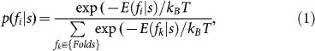where kB is the Boltzmann constant and T is the temperature. The denominator is the partition function Z, which sums the probability of s over all the folds considered. kB is set to 115,18. As the set of the possible folds, we considered all the 41 compact folds that fit in the 4 × 4 square as well as semi-compact folds that fit within 5 × 5 (493 folds) or 6 × 6 squares (1588 folds). We consider that the compact (or semi-compact) folds correspond to native folds of proteins, since usually native folds have well-defined tertiary structures as opposed to unfolded states of proteins. We suppose that the compact (or semi-compact) native folds carry out essential functions of an organism20, which are needed for sustaining life. Thus, an organism needs to code all of the folds in its genome sequence in an energy-efficient manner. To this end, we investigated how the essential folds can be coded efficiently by a genome sequence (i.e. a set of amino acid sequences) of an organism and its outcome in terms of the sequence and fold distribution. By efficient coding, we mean that the information of folds possessed by the sequences is maximized.

This question can be readily rephrased in information theoretic terms using the concept of the capacity of a noisy channel13. More concretely, a fundamental question regarding communication over a noisy channel is the following: what is the maximum amount of information per input symbol that can be reliably transmitted? This maximum transmission rate C is called the capacity of the channel. It turns out that C is equal to the maximum, taken over all possible input distributions, of a quantity called the mutual information I(S; F) between the input S and output F, i.e. C = maxp(s)I(S; F). The mutual information between two random variables can be thought of as a measure of the statistical dependence between them. It is given by I(S; F) = H(F) - H(F|S), where H(F) and H(F|S) are the entropy of F and conditional entropy of F given S, respectively. In this study F corresponds to the set of protein folds and S is the set of sequences. When a sequence s folds into f with a probability of p(f|s), we would like to know the sequence distribution that maximizes the mutual information with all the necessary protein folds, i.e. the sequence distribution that is almost achieving the channel capacity. The channel, which we call the protein sequence-fold channel, is illustrated in Figure 1. The channel is defined by p(f|s), the Boltzmann distribution, which takes an input sequence distribution and outputs a fold distribution. The mutual information I(S; F) was maximized using the Arimoto-Blahut algorithm21,22, which iteratively increases I(S; F) by revising the sequence distribution (Materials and Methods) and reports the resulting mutual information and distributions of sequences and folds. Practically, the algorithm was run until the increase of I(S; F) by an iteration was sufficiently small (see Figure 2 caption).

### Sequence distribution that nearly achieve channel capacity

The resulting sequence distributions that nearly achieve the channel capacity are shown in Figure 2. Two temperatures T were used for computing the Boltzmann distribution: one that is equal to or less than the folding temperature18 Tf of 50% of the sequences while another one is set to lower than the former such that it is equal to or less than Tf of 80% of the sequences. Tf for a protein sequence s is defined as the largest temperature where the native (most dominant) fold fnative shares over 50% of the probability, i.e. P(fnative|s) ≥ 0.518. Tf is different in principle for each sequence. To compute the two temperatures, only sequences that have a unique dominant fold were considered. The fraction of sequences whose Tf is equal to or lower than each temperature was plotted (Supplementary Figure 1).

Figure 2A is the resulting histogram of the fraction of sequences that have each given probability P(s) when the 41 compact folds that fit within the 4 × 4 lattice were considered. The distribution of fractions of sequences is highly skewed for both temperatures. Using the temperature 1.26 (0.67), 99.6 (97.1)% of sequences having a probability below 10−4 while only 0.18 (0.28)% of sequences have a probability over 10−3. In each case the distribution follows a power law, i.e. Fraction(P(s)) P(s)−γ with γ = 0.92 (1.48). (In the parentheses, values for T = 0.67 were shown.) The overall trend does not change when we also take semi-compact folds into consideration (Figs. 2B, 2C). The sequence distributions for folds that fit to 5 × 5 and 6 × 6 clearly follow a power law with the degree exponent (γ) of 1.06 (1.42) and 1.07 (1.36) for 5 × 5 and 6 × 6, respectively. (In the parentheses, values for a lower temperature were shown.)

The histogram of sequence fractions remains skewed at different high temperatures up to 20 (Fig. 2D). At even higher temperatures (T = 50, 75, 100, 150 and 200 were tested), the distribution became flat with all the sequences having almost the same probability, since probability of folds for a sequence will become less distinguishable between each other. On the other hand, the mutual information I(S; F) showed a two state transition as the temperature increases (Fig. 2E)23. At the temperature of around 1.1 to 1.2, which is slightly higher than Tf, I(S; F) decreases to almost to 0 since all the sequences have almost equal probability.

The results in Figure 2A–C indicate that a set of indispensable protein folds are most efficiently coded when the histogram of sequences follows a power law distribution. As a probability assigned to each HP sequence in our model can be interpreted as the population that the sequence shares, an HP sequence would corresponds to a protein family in nature. Indeed, the sequence histogram observed for the lattice models closely resembles the distribution of actual protein sequence families. Figure 2F shows the distributions of superfamilies that belong to each fold in the CATH protein structure classification database. It follows a power law distribution with the degree exponent values γ = 1.24. The power law distribution of protein families shown here is consistent with what was previously reported1,2.

### Protein fold distribution

Next, having discussed the nearly capacity-achieving sequence distribution obtained from the Arimoto-Blahut algorithm, we ask what the corresponding fold distribution looks like. This distribution, in which the probability of a fold is calculated by conditioning on sequences (i.e., where N is the total number of sequences), does not exhibit a power law (Supplementary Figure 2); rather, it is somewhat close to a uniform distribution. This phenomenon may be explained if we consider that the mutual information is defined as I(S; F) = H(F) − H(F|S), which can be maximized by having a fold distribution as uniform as possible. However, the folds do exhibit a power law distribution when each sequence s is assigned with a single fold that has the maximum probability according to the Boltzmann distribution (i.e.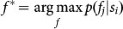) (Fig. 3A). Skewness of the distributions is clear for folds within 5 × 5 and 6 × 6, which include a large enough number of folds. Assigning a single fold to a sequence would be more natural, because in general a protein is folded into its lowest free energy19. The fold distribution shown in Figure 3A is consistent with actual protein folds in nature1,5 (Fig. 3B) as well as those shown in previous theoretical studies10,11. Thus, importantly, the origins of the power-lawness for the sequence and the fold distribution are suggested to be different; the former comes from the information theoretic nature of the sequence-fold channel while the latter comes from thermodynamics of proteins.

### Fold duplicator channel that achieves a power-law distribution for folds

Knowing that the protein folds exhibit a power law from their thermodynamical nature but not from a direct outcome of the information theoretic protein sequence-fold channel, we next modified the channel such that the fold distribution follows a power law and reexamined the near capacity-achieving sequence distribution. In the modified channel, individual instances of arbitrarily selected folds are multiplicated by cascading the original channel with a new duplicator channel that maps each fold f to elements in the set Fold'(f) according to a certain conditional distribution (Fig. 1B) with two important properties: distinct folds f1 and f2 map to disjoint sets (i.e. Fold'(f1) and Fold'(f2) share no elements) and each set Fold'(f) has elements with conformation identical to that of f. To realize a power law distribution, the size of each Fold'(f) is chosen so that most sets are small, while a few are large. We proved a proposition stating that the capacity and the optimal sequence distribution are exactly the same in the original sequence-fold channel (Fig. 1A) and the modified sequence-fold-duplicator channel, regardless of choices of sizes of the Fold' sets and of conditional distributions. The mathematical proof is shown in Supplemental Material. Thus, even when we require a power law fold distribution, the optimal sequence distribution remains skewed.

### Characteristics of sequences and folds in the capacity-achieving channel

In the near capacity-achieving sequence histogram, folds coded by a sequence with a large probability P(s) also have a high probability, which indicates that well populated sequences code more stable folds. To illustrate this, we show the conditional probability of folds for the ten most populated sequences (Figs. 4A, 4B) and for the ten least populated sequences (Figs. 4C, 4D) for the case of the 5 × 5 lattice with T = 0.45 as an example. The fold distributions of the ten most and least populated sequences for all 4 × 4, 5 × 5 and 6 × 6 lattices are further provided in the supplemental material (Supplementary Figure 3). There is a striking difference between fold distributions coded by the most and least probable sequences: The most populated sequences code a dominant fold that has a high probability of 0.8 to 1.0 (Figs. 4A, 4B). In contrast, the least populated sequences do not have a single fold with a distinctively high probability; rather they have multiple folds with an equal, small probability, which often cover almost the entire fold space (Figs. 4C, 4D). This trend holds for the compact (4 × 4) and semi-compact (5 × 5 and 6 × 6) folds. The results imply that the nature removes protein sequences which ambiguously code many different structures with the same probabilities. Figure 4 and Supplementary Figure 3 show limited examples but the positive correlation between the probability of sequences and that of folds coded by the sequences was observed for the entire sequences (Supplementary Fig. 4). This observation is consistent with what was reported by Sali et al.16 and a recent work which reports that highly abundant proteins favor more stable 3D structures in a genome24. By visual inspection of high- and low-probability sequences and their folds we found that folds with a high probability have a hydrophobic core while low-probability sequences are dominated by either one of the amino acid types (H, P) or H and P come one after another and unable to make energetic distinction between folds. In Figure 5, we show folds coded by the ten highest and lowest populated sequences for the 5 × 5 lattice when T = 0.45. Folds coded by ten highest and lowest populated sequences for the 4 × 5, 5 × 5 and 6 × 6 lattices are further provided in Supplementary Figure 5.

## Discussion and Conclusions

The hypothesis that sequences are chosen in a way so as to achieve the capacity of the sequence-to-structure channel explains the skewed, power law character of the sequence distribution as observed in nature, even when external conditions constrain the fold distribution to also follow a power law. Previous works proposed mathematical evolutionary models that result in power law behavior of protein sequences1,25,26,27, without explaining why such mechanisms are beneficial. The current work provides an explanation why it is beneficial – it can maximize information of folds contained per sequence unit (e.g. amino acid). Another important conclusion is that the origin of the power law distribution is suggested to be different for sequences and folds: protein sequences exhibit a power law distribution to achieve efficient coding of necessary folds, whereas the power lawness of the fold distribution can be attributed to the Boltzmann distribution of energy levels of folds for each sequence. The results may also suggest that power law distributions observed in various biological instances, including protein families1,2,27, domains25, folds1,5 and networks28,29, may be of different origins.

## Methods

### Channel Capacity Computation with Arimoto-Blahut algorithm

We explain how the capacity-achieving distribution for the sequence-to-structure channel is computed. The capacity C of the channel, as well as the optimizing sequence distribution Pr*(S), is computed using the Arimoto-Blahut algorithm, whose inputs are the channel and an ε > 0 whose purpose is to determine the termination condition of the algorithm. Roughly speaking, the key insight is that the original optimization problem can be reformulated in terms of two variables whose values can easily be alternatingly optimized until a desired level of convergence is achieved.

We define the objective function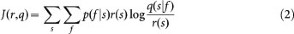In the above, p(f|s) is the probability of fold f given sequence s, which is given by the channel.

The variable r(s) is the probability of sequence s and q is a conditional distribution over sequences given folds.

For a fixed input distribution r, the maximum of J with respect to q is attained whenFor a fixed q, it can be shown that the maximizing value of r is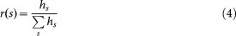The capacity can then be shown to be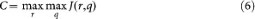The core of the algorithm is as follows.

1. Choose an initial guess r0 for the optimizing sequence distribution. Execute the following steps to compute r1, r2, … rt, where rt is the first r such that the Euclidean metric d(rt, rt−1) ≤ ε. In what follows, the current iteration number is given by k.

2. Maximize J(rk, q) with respect to q according to (2) to get qk.

3. Maximize J(r, qk) with respect to r according to (1) to get rk+1.

From Pr*(S) and the channel, the optimizing distribution over structures is given by, for each f Fold,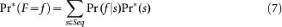## References

• Qian, J., Luscombe, N. M. & Gerstein, M. Protein family and fold occurrence in genomes: power-law behaviour and evolutionary model. J. Mol. Biol. 313, 673–681 (2001).

• Enright, A. J., Kunin, V. & Ouzounis, C. A. Protein families and TRIBES in genome sequence space. Nuc Acids Res. 31, 4632–4638 (2003).

• Abeln, S. & Deane, C. M. Fold usage on genomes and protein fold evolution. Proteins 60, 690–700 (2005).

• Koonin, E. V., Wolf, Y. I. & Karev, G. P. The structure of the protein universe and genome evolution. Nature 420, 218–223 (2002).

• Orengo, C. A., Jones, D. T. & Thornton, J. M. Protein superfamilies and domain superfolds. Nature 372, 631–634 (1994).

• Wolf, Y. I., Grishin, N. V. & Koonin, E. V. Estimating the number of protein folds and families from complete genome data. J. Mol. Biol. 299, 897–905 (2000).

• Yan, Y. & Moult, J. Protein family clustering for structural genomics. J. Mol. Biol. 353, 744–759 (2005).

• Oberai, A., Ihm, Y., Kim, S. & Bowie, J. U. A limited universe of membrane protein families and folds. Protein Sci. 15, 1723–1734 (2006).

• Liu, X., Fan, K. & Wang, W. The number of protein folds and their distribution over families in nature. Proteins 54, 491–499 (2004).

• Zeldovich, K. B., Berezovsky, I. N. & Shakhnovich, E. I. Physical origins of protein superfamilies. J. Mol. Biol. 357, 1335–1343 (2006).

• Li, H., Helling, R., Tang, C. & Wingreen, N. Emergence of preferred structures in a simple model of protein folding. Science 273, 666–669 (1996).

• Finkelstein, A. V. & Ptitsyn, O. B. Why do globular proteins fit the limited set of folding patterns? Prog. Biophys Mol Biol 50, 171–190 (1987).

• Cover, T. M. & Thomas, J. A. Elements of Information Theory 2nd Edition. (John Wiley & Sons, 2006).

• Anfinsen, C. B. Principles that govern the folding of protein chains. Science 181, 223–230 (1973).

• Sali, A., Shakhnovich, E. & Karplus, M. Kinetics of Protein-Folding - a Lattice Model Study of the Requirements for Folding to the Native-State. J Mol. Biol. 235, 1614–1636 (1994).

• Sali, A., Shakhnovich, E. & Karplus, M. How does a protein fold? Nature 369, 248–251 (1994).

• Lau, K. F. & Dill, K. A. Theory for protein mutability and biogenesis. PNAS 87, 638–642 (1990).

• Nakamura, H. K. & Sasai, M. Population analyses of kinetic partitioning in protein folding. Proteins 43, 280–291 (2001).

• Shakhnovich, E. I. & Gutin, A. M. Implications of thermodynamics of protein folding for evolution of primary sequences. Nature 346, 773–775 (1990).

• Finkelstein, A. V., Gutun, A. M. & Badretdinov, A. Why are the same protein folds used to perform different functions? FEBS letters 325, 23–28 (1993).

• Blahut, R. E. Computation of channel capacity and rate-distortion functions. IEEE Trans. Inform. Theory 18, 460–473 (1972).

• Arimoto, S. An algorihtm for computing the capacity of arbitrary discrete memoryless channels. IEEE Trans. Inform. Theory 18, 14–20 (1972).

• Magner, A., Kihara, D. & Szpankowski, W. Phase transitions in a protein folding channel. Information Theory and Applications Workshop, La Jolla, San Diego, CA. (2015).

• Serohijos, A. W., Lee, S. Y. & Shakhnovich, E. I. Highly abundant proteins favor more stable 3D structures in yeast. Biophys J. 104, L1–3 (2013).

• Molina, N. & van Nimwegen, E. The evolution of domain-content in bacterial genomes. Biology Direct 3, 51 (2008).

• Karev, G. P., Wolf, Y. I., Rzhetsky, A. Y., Berezovskaya, F. S. & Koonin, E. V. Birth and death of protein domains: a simple model of evolution explains power law behavior. BMC Evol Biol 2, 18 (2002).

• Huynen, M. A. & van Nimwegen, E. The frequency distribution of gene family sizes in complete genomes. Mol Biol Evol 15, 583–589 (1998).

• Jeong, H., Tombor, B., Albert, R., Oltvai, Z. N. & Barabasi, A. L. The large-scale organization of metabolic networks. Nature 407, 651–654 (2000).

• Yook, S. H., Oltvai, Z. N. & Barabasi, A. L. Functional and topological characterization of protein interaction networks. Proteomics 4, 928–942 (2004).

## Acknowledgements

Yifeng D. Yang's contribution to an early stage of this work is acknowledged. This work has been supported in parts by grants from the National Institutes of Health (R01GM097528), National Science Foundation (IIS1319551, DBI1262189, & IOS1127027). and National Research Foundation of Korea (NRF-2011-220-C00004) to DK. WS and AM have been supported by NSF Center for Science of Information (CSoI) Grant CCF-0939370 and in addition by NSA Grant 130923 and DMS-0800568 and the MNSW grant DEC -2013/09/B/ST6/02258. WS is also with the Faculty of Electronics, Telecommunications and Informatics, Gdansk University of Technology, Poland.

## Author information

Authors

### Contributions

A.M. coded programs, performed computational analyses and participated in writing the paper. A.M. and D.K. prepared figures. W.S. participated in interpreting results and participated in proving mathematical propositions. D.K. conceived and designed the study and wrote the paper. All authors read and approved the manuscript.

## Ethics declarations

### Competing interests

The authors declare no competing financial interests.

## Electronic supplementary material

### Supplementary Information

Supplemental Material

## Rights and permissions

Reprints and Permissions

• ### A Dynamic Model for the Evolution of Protein Structure

• Guy Tal
• Simina Maria Boca
• Gustavo Caetano-Anollés

Journal of Molecular Evolution (2016)